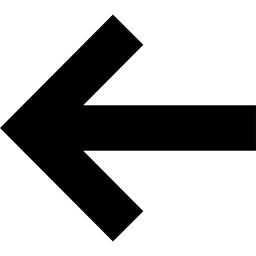# Quantum Number (QN) Objects

As shown in examples on the previous pages, subspaces of a QN Index are labeled by QN objects, such as QN("Sz",1) or QN({"N",2},{"Sz",0}). This page goes into more detail about how these QN objects work, what mathematical rules they obey, and how to construct them.

From a physics perspective, quantum numbers are quantities which are conserved under the dynamics of a system. Examples include total particle number, particle number modulo 2, or total z-axis magnetization. Mathematically, quantum numbers arise from the representation theory of Abelian groups, which relates their irreducible representations to products of $\mathbb{Z}$ or $\mathbb{Z}_n$ .

## QN Object Basics

At the simplest level, a QN is a small set of quantum numbers stored as name-value pairs, where the name is a short string of up to seven characters and the value is an integer.

For example, a QN constructed as QN("N",2) contains one quantum number named "N" with the value 2.

A QN constructed as QN({"Nb",1},{"Sz",2}) contains two quantum numbers: one named "Nb" with the value 1, the other named "Sz" of value 2.

QN objects can be added, subtracted, and compared to each other. For QNs like those above, addition and subtraction follows the usual rules of integer arithmetic. Quantum numbers with the same name are added to each other. If a QN contains a quantum number which is not present in another QN, such a case is treated as if the other QN did have that quantum number but with the value equal to zero. This rule about missing quantum numbers being treated as zero applies to addition, subtraction, and comparison.

For example: QN({"N",3}) + QN({"Sz",2},{"N",1}) == QN({"Sz",2},{"N",4}).

As another example, QN({"N",3}) == QN({"Sz",0},{"N",3}) compares true.

The quantum numbers within a QN are not ordered, from a user perspective. Internally they are sorted by name, but you may provide them in any order and the QN system will handle the sorting for you.

Besides regular integer arithmetic, arithmetic modulo N (according to the group $Z_N$ ) can also be defined for QN—we will discuss this case more below.

## Special Cases of the QN Constructor

Note that when constructing a QN containing just one quantum number, it is allowed to omit the curly braces: QN("Sz",2).

Also, at most one of the quantum numbers in a QN can be unnamed, which is equivalent to letting the name be the empty string "". This is for convenience when no name is obvious. For example, QN(0) and QN(1) are valid QNs and equivalent to QN({"",0}) and QN({"",1}).

## Modular Arithmetic

An important case of quantum numbers for physics applications are quantum numbers obeying a $Z_N$ addition rule. Key examples include fermion parity obeying a $Z_2$ addition rule or clock-spin models obeying a $Z_3$ addition rule.

To specify $Z_N$ addition for one or more quantum numbers within a QN, simply specify the modulus $N$ as a third entry when defining that quantum number. For example, QN("P",0,2) and QN("P",1,2) defines a quantum number named "P" (for parity) which obeys a $Z_2$ addition rule, and which takes the values 0 and 1 in the respective QNs constructed. Thus QN("P",1,2)+QN("P",1,2) == QN("P",0,2).

Another example would be QN({"Sz",1},{"T",2,3}) which defines a quantum number named "Sz" with value 1 obeying usual integer arithmetic, whereas the quantum number named "T" has value 2 and obeys a $Z_3$ addition rule.QN IndexBack to BookBack to Main